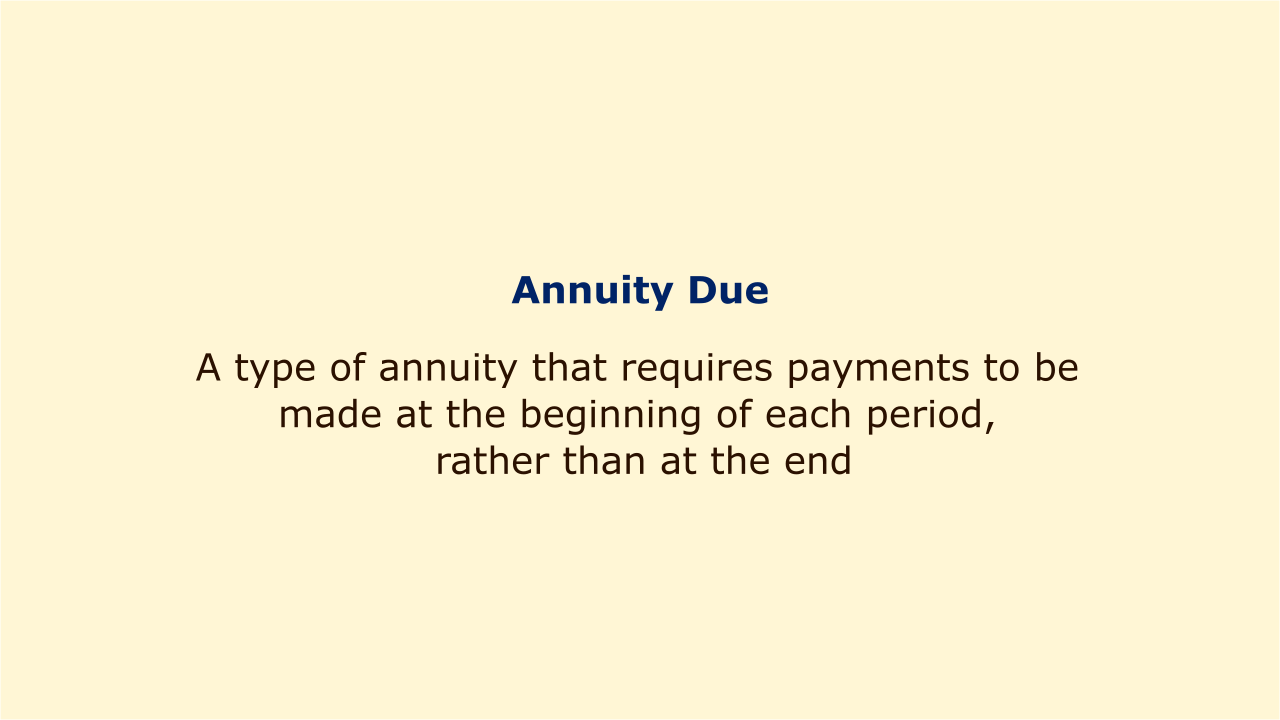# Annuity DueImage: Moneybestpal.com

### Annuity Due is a type of annuity that requires payments to be made at the beginning of each period, rather than at the end. Rentals, leases, insurance payments, and various kinds of retirement plans frequently use Annuity Due. An alternative to Annuity Due is Regular Annuity, which is more typical for loans and mortgages.

The timing of the installments distinguishes Annuity Due from Regular Annuity the most. Annuity Due payments are made sooner, giving them more time to amass interest and increase in value. As a result, although if an Annuity Due has the same payment amount, interest rate, and number of periods as an Ordinary Annuity, its present value and future value are higher.

To calculate the present value and future value of an Annuity Due, we can use the following formulas:

Present Value of Annuity Due = PMT x [(1 - 1/(1 + r)^n) / r] x (1 + r)

Future Value of Annuity Due = PMT x [(1 + r)^n - 1] / r x (1 + r)

Where:

PMT = Periodic payment amount

r = Periodic interest rate

n = Number of periods

Alternatively, we can use the formulas for Ordinary Annuity and multiply them by (1 + r) to get the same results.

#### Let's look at some examples of how to use these formulas.

Example 1: You want to spend \$25,000 on a vehicle. You have two payment options: \$500 monthly for 60 months at the end of each month (Ordinary Annuity) or \$500 monthly for 60 months at the start of each month (Annuity Due). The interest rate is 6% annually, compounded every month. Which option is cheaper for you?

Solution: To compare the two options, we need to find the present value of each payment stream.

For Ordinary Annuity:

Present Value = \$500 x [(1 - 1/(1 + 0.06/12)^60) / (0.06/12)]

Present Value = \$24,465.88

For Annuity Due:

Present Value = \$500 x [(1 - 1/(1 + 0.06/12)^60) / (0.06/12)] x (1 + 0.06/12)

Present Value = \$25,933.83

As you can see, the present value of the Annuity Due is more than the present value of the Regular Annuity, making it more expensive for you to pay at the start of each month. The Ordinary Annuity choice is what you should pick.

Example 2: You decide to set aside \$2,000 annually for 30 years in an account that offers 8% interest, compounded annually, in order to save for retirement. Either at the beginning of the year (Ordinary Annuity) or at the end of the year, you can invest (Annuity Due). After 30 years, how much will each of you have in your account?

Solution: To compare the two options, we need to find the future value of each payment stream.

For Ordinary Annuity:

Future Value = \$2,000 x [(1 + 0.08)^30 - 1] / 0.08

Future Value = \$251,566.42

For Annuity Due:

Future Value = \$2,000 x [(1 + 0.08)^30 - 1] / 0.08 x (1 + 0.08)

Future Value = \$271,691.74

As you can see, if you invest at the beginning of every year, Annuity Due will have a higher future value than Regular Annuity, resulting in a larger balance in your account. The Annuity Due option is the one you should pick.
Tags